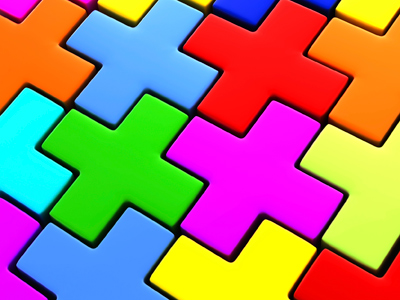Addition, plus, more than, total, altogether and sum are all words which mean add.

This Math quiz is called 'The Language of Addition' and it has been written by teachers to help you if you are studying the subject at elementary school. Playing educational quizzes is a fun way to learn if you are in the 1st or 2nd grade - aged 6 to 8.

It costs only \$12.50 per month to play this quiz and over 3,500 others that help you with your school work. You can subscribe on the page at Join Us

In elementary school, children extend their understanding of the language of addition to include the word sum. They should already know words such as add, addition, plus, more than, total and altogether. Recognizing and understanding the language of addition is essential in order to be able to make decisions about which operation to use to solve calculations and problems.

Can you recognize the addition words?

1.
38 is the total of which 2 numbers?
80 and 3
20 and 4
30 and 8
3 and 8
To find the total, add all the numbers together
2.
What is the symbol for addition?
-
+
=
÷
3.
What is the sum of 7, 9 and 3?
17
23
18
19
To find 'the sum' add all the numbers together
4.
Which word could be used in this number sentence?
9 ___ 6 = 15
take away
subtract
divided by
To add means to count up the total of all the numbers together
5.
15 is the sum of which 2 numbers?
6 and 9
11 and 3
15 and 4
9 and 8
The sum of the numbers is what you find when they are added together
6.
How much is 6 more than 8?
28
68
12
14
'More than' means counting on from one number
7.
Which word could be used in this number sentence?
7 ___ 5 = 12
plus
minus
subtract
multiplied by
8.
Which of the following is not an addition word?
Sum
Division
Altogether
Total
Division is not adding or finding a total or sum
9.
What is the total of 12, 3 and 5?
25
18
20
19
To find the total, add all the numbers together
10.
I have 4 cakes, 5 buns and 2 cookies. How many treats do I have altogether?
7
9
11
15
The clue word in the problem is 'altogether'
Author:  Angela Smith